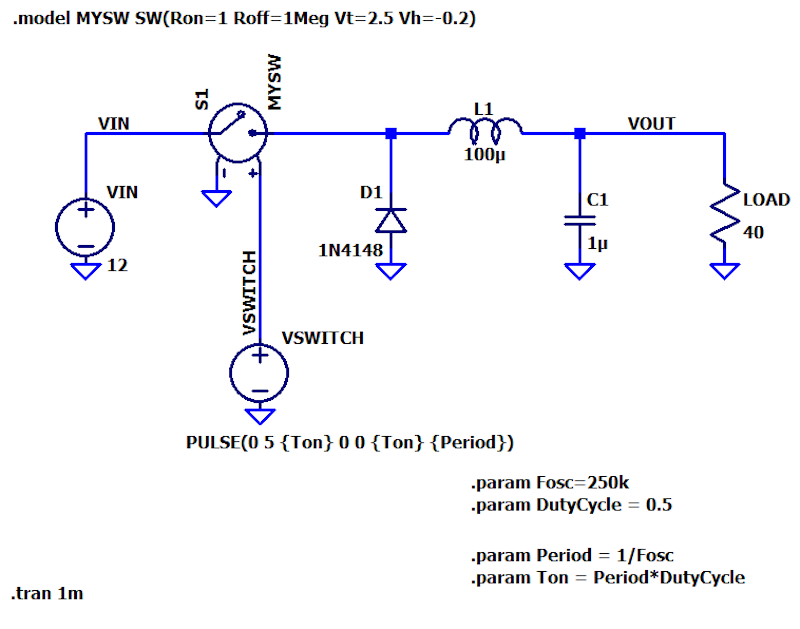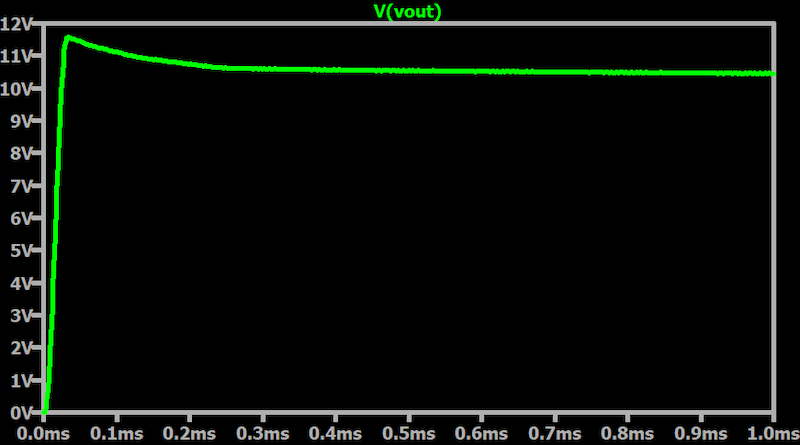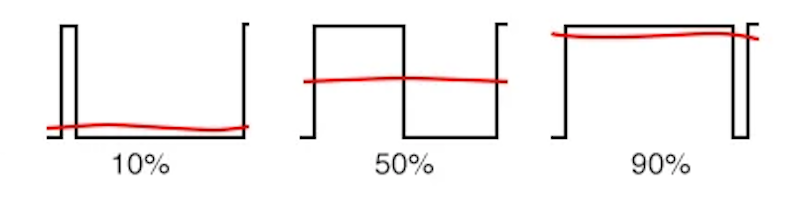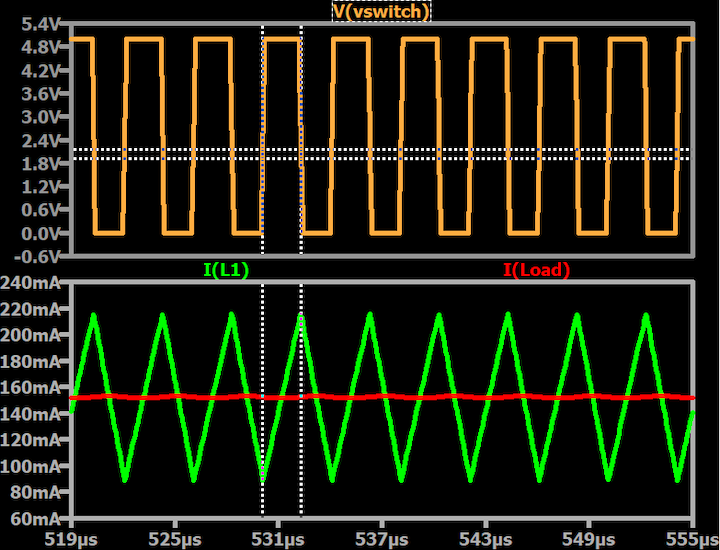Technical Article

# Understanding Switch-Mode Regulation: The Buck Converter

July 30, 2023 by Robert Keim

## This article will analyze in detail the operation of a step-down DC/DC voltage converter. Using SPICE simulations, we will investigate the output voltage settling, voltage ripple, and inductor and load currents.

I previously provided a high-level conceptual overview of switch-mode voltage regulation. I’d like to follow that theoretical treatment with a thorough examination of the current and voltage behavior using LTSpice to simulate a switch-mode buck converter.

### Buck (Step-Down) Converter Design

The circuit that we’ll be working with is illustrated in the schematic of Figure 1. This is known as a buck or step-down converter.##### Figure 1. Buck (step-down) converter simulation schematic

The buck or step-down converter can be used to accomplish a common task for power-management circuits: reducing a standard system-level voltage, such as 12 or 28 V, to a 5 or 3.3 V supply rail that is appropriate for low-voltage electronics.

I say “help” because the topology of Figure 1 is only the power stage of a switch-mode regulator. It is not a complete regulator because it lacks feedback and, therefore, cannot lock onto a specified output voltage.

### Buck Converter Schematic and Simulation Details

Before we dive into the simultion and analysis, let's discuss a few of the less self-explanatory aspects of this LTspice schematic:

• The power switch model
• The switching frequency and duty cycle
• One implication of using an ideal voltage source at the input
• The selection of the inductor and capacitor values

#### The Power Switch Model

The power switch in physical converter circuits is usually a field-effect transistor. In this simulated circuit, I’m using a voltage-controlled switch whose specifications are determined by the .model MYSW SW(...) statement. The switching characteristics are highly favorable but not quite ideal:

• 1 Ω on-state resistance
• 1 MΩ off-state resistanc
• A threshold voltage right in the middle of the logic-low (0 V) and logic-high (5 V) levels generated by VSWITCH.

#### The Switching Frequency and Duty Cycle

VSWITCH produces a rectangular wave that turns the switch on and off. Using the .param statement, I’ve defined various parameters that allow me to easily control the key switching characteristics. I specify the oscillator frequency and duty cycle, which are the values required by my brain to think intuitively about circuit behavior. These are used to calculate the period and on time, which are the values required by LTspice’s PULSE function.

#### Simulation Using an Ideal Voltage Source at the Input

The output capacitor is integral to converter operation and is, therefore, necessary in both simulated and physical circuits. Physical circuits also need an input capacitor, which serves the critical purpose of reducing source impedance and thereby allowing the converter to more smoothly draw intense bursts of current from the input supply. Since the input supply in my SPICE implementation has zero series impedance, no input capacitor is needed.

#### The Selection of the Inductor and Capacitor Values

The inductance (100 μH) and capacitance (1 μF) values shown in Figure 1 are reasonable starting points that I calculated using equations found in this TI app note. We’ll explore the effects of capacitor and inductor values in a future article.

### Buck Converter Simulation

Let’s start by running a simulation with a 50% duty cycle and a load resistance of 1 kΩ. Figure 2 is a plot of the output voltage over time. Note that the output voltage needs some time to reach its steady-state value.##### Figure 2. Buck converter output voltage settling.

Switch-mode converters, including the capacitor-based switchers that we call charge pumps, have a start-up delay corresponding to the amount of time needed to charge the output capacitor. This occurs in pretty much any circuit, as there’s always some capacitance somewhere that needs to be charged.

With a switcher, though, start-up time can be considerably longer because the charging current is limited by the switching action and because the amount of capacitance to be charged is relatively large.

### The Effect of Duty Cycle on the Buck Converter Output Voltage

As illustrated in Figure 2, with an input voltage is 12 V, the steady-state output voltage is about 10.5 V. The duty cycle is 50%, so why is the output voltage so much higher than 50% of the input voltage?

If you have read the previous article, you saw the diagram (repeated here in Figure 3) in which the magnitude of a filtered voltage corresponds directly to the duty cycle of a PWM waveform.##### Figure 3. DC voltage levels as a function of the PWM duty cycle.

However, this diagram depicts only the effect of filtering a PWM waveform. whereas in a switch-mode converter, the PWM duty cycle is just one of the various factors that influence the VIN-to-VOUT ratio. I can leave the duty cycle at 50% and significantly change the output voltage by modifying the value of the inductor, the amount of load resistance, or the switching frequency.

This discussion serves as a good reminder that the duty cycle is not a means of generating a specific, fixed output voltage. Rather, switch-mode regulators achieve stable output voltage by adjusting the duty cycle as part of a closed-loop control scheme made possible by negative feedback.

Thus, we can’t just set the duty cycle at 50% and assume that we’ll have VOUT = 6 V with VIN = 12 V. In any case, we don’t want to build a regulator around a constant input voltage. We would much prefer to design a regulator that is robust against unexpected input variations and readily integrated into new applications that may have different input voltages.

Before concluding this section, I want to briefly mention that PWM is not the only way to perform switch-mode regulation. Though less common, pulse-frequency modulation (PFM) is preferred in some applications because it offers enhanced efficiency in light-load conditions.

### Buck Converter Inductor Current

We’ll conclude with the plot of Figure 4 which shows the inductor current relative to the switch-control voltage and the output current.##### Figure 4. Buck converter switching voltage (top), inductor current (bottom-green), and load current (bottom-red).

The plot of switch-control voltage conveys the state of the switch: it’s open when the switch-control signal is logic low and closed when the signal is logic high. In accordance with the fundamental principle of switch-mode regulation, the switch is always driven fully on or fully off, meaning that it is either freely passing current or completely blocking current.

Unmodified, this sort of on/off current cannot properly power electronic circuitry, but the plot of the inductor current shows the ramp-up/ramp-down effect described in the previous article. The current through an inductor can’t change instantaneously, and the topology of the buck converter allows the inductor to convert on/off current into ramp-up/ramp-down current. As indicated by the dotted cursor lines, the inductor current starts ramping up when the switch turns on and starts ramping down when the switch turns off.

The inductor current alone would have too much ripple for power-supply purposes. However, the inductor works in conjunction with the output capacitor, which provides enough filtering to enable the steady, low-ripple load current that you see in the plot. Note that the load current is the average value of the inductor current.

### Application of Buck Converters

The buck converter is commonly used in power-management systems to generate the lower DC voltages used in modern electronics. We’ve examined some key aspects of switch-mode DC voltage converter operation using LTspice.

Hopefully, understanding the basic operation of this simplified schematic will assist you in your circuit design efforts. In the next article, we’ll carefully analyze current and voltage behavior during the switch-on state and the switch-off state.